Function Repository Resource:

# NumberOfTableaux

Get a count of Young tableaux for a given size or shape

Contributed by: Ed Pegg Jr and Steven Skiena
 ResourceFunction["NumberOfTableaux"][t] returns the number of Young tableaux with shape t, where the shape is a decreasing integer partition. ResourceFunction["NumberOfTableaux"][n] returns the total number of Young tableaux for all decreasing integer partitions of n.

## Details

A Young tableau is a list of lists of decreasing length partitioning a permutation. For all entries in a Young tableau, t[[a,b]]<t[[a+1,b]] and t[[a,b]]<t[[a,b+1]].
Young tableaux are often called tableaux.
A few pictures for items related to Young tableaux: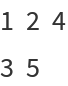Young diagram of Young tableau {{1,2,4},{3,5}}, English notation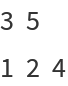Young diagram of Young tableau {{1,2,4},{3,5}}, French notation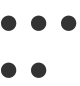Ferrers diagrams of Young tableau {{1,2,4},{3,5}} {{1,2,4},{3,5}} Young tableau with shape {3,2}

## Examples

### Basic Examples (1)

Count the tableaux with shape {3,2}:

 In:=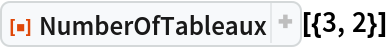Out=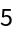### Scope (2)

Find the integer partitions of 6:

 In:=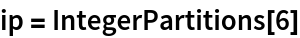Out=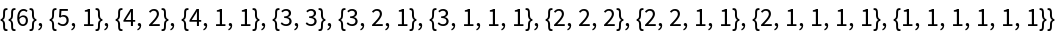Using the partitions as shapes, find the tableaux count for each shape:

 In:=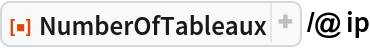Out=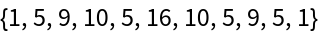Calculate the total:

 In:=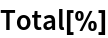Out=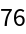Find the number of tableaux with order 6:

 In:=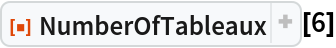Out=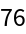Determine the shape of an order-15 tableau:

 In:=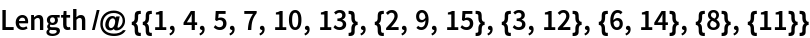Out=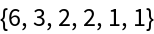Count tableaux with that shape:

 In:=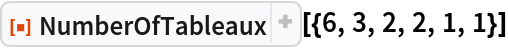Out=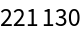## Version History

• 1.0.0 – 24 January 2022